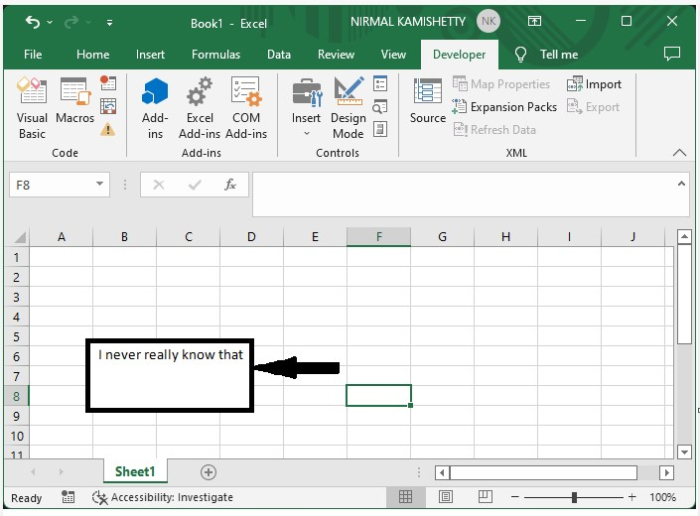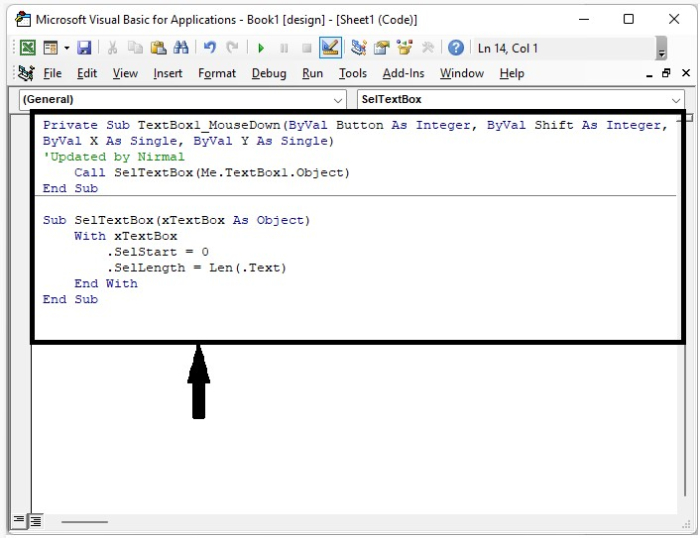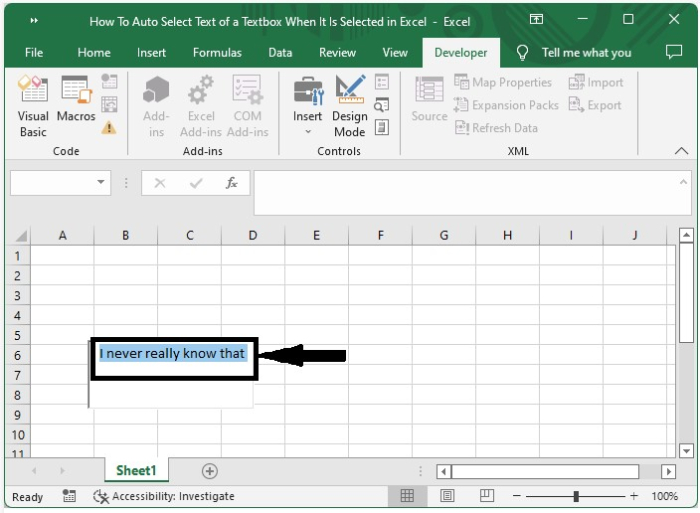# How to Auto-Select the Text of a Textbox When It is Selected in Excel?

When we are dealing with text boxes, it is very important to update and delete the data inside them, but if it contains a large amount of data, we need to select the data inside the text box and delete it. In this tutorial, I will explain the process for selecting the data in a text box by clicking on it. This tutorial will help you understand how you can autoselect text from a textbox when it is selected in Excel.

## Auto-Select the Text of a Textbox When It is Selected

Here, we will first create a macro and then assign it to the text box. Let us see a simple process to know how we can auto-select text from a textbox when it is selected in Excel using the VBA application. We will be using an ActiveX command textbox under the developer option.

Step 1

Let us consider an Excel sheet where an active "X" command text box is present, similar to the sheet shown in the below image.To open the VBA application, right-click on the text box and select View Code, then type the programme into the text box as shown in the image below.

### Example

Private Sub TextBox1_MouseDown(ByVal Button As Integer, ByVal Shift As Integer, _
ByVal X As Single, ByVal Y As Single)
'Updated by Nirmal
Call SelTextBox(Me.TextBox1.Object)
End Sub

Sub SelTextBox(xTextBox As Object)
With xTextBox
.SelStart = 0
.SelLength = Len(.Text)
End With
End SubStep 2

Now save the sheet as a macro-enabled sheet and close the vba application using the command "Alt + Q", which then exits the designer mode, and every time we click on the textbox, all the data in it will be selected as shown in the below image.If you have multiple textboxes, then you can add the following code to the program.

### Example

Private Sub TextBox2_MouseDown(ByVal Button As Integer, ByVal Shift As Integer, ByVal X As Single, ByVal Y As Single)
Call SelTextBox(Me.TextBox2.Object)
End Sub


## Conclusion

In this tutorial, we used a simple example to demonstrate how you can auto-select the text from a textbox when it is selected in Excel.